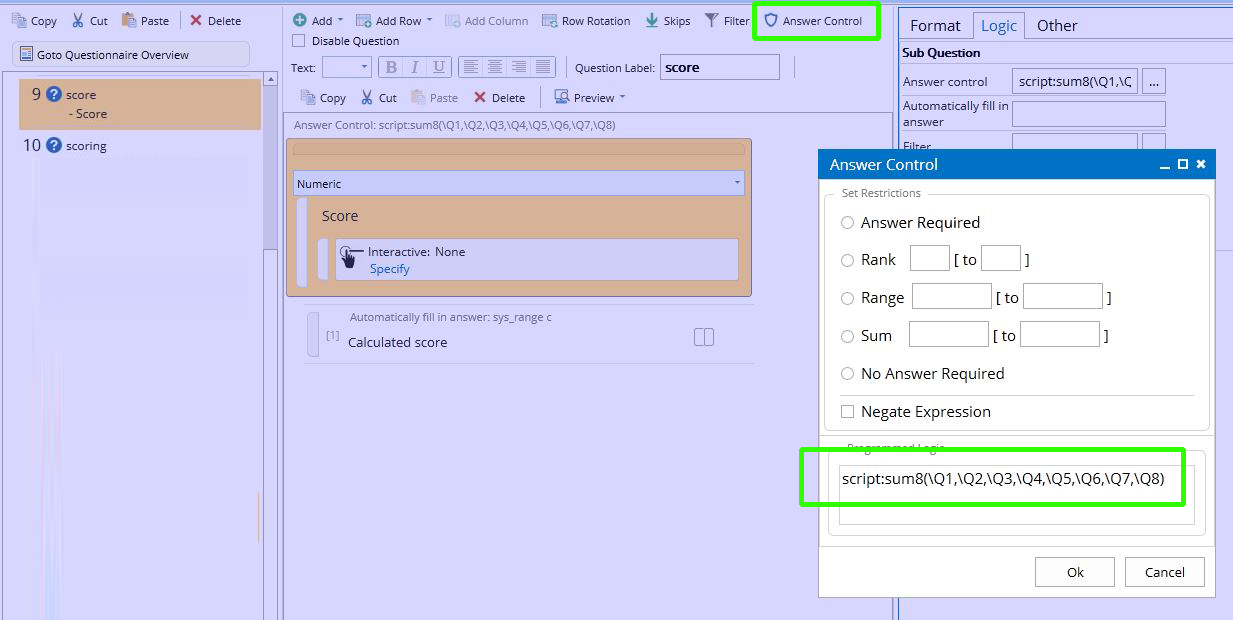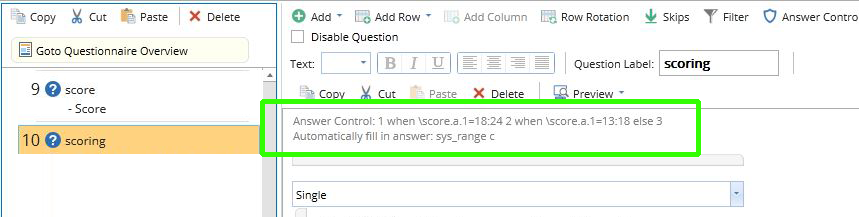# Sum

Description:

script:sumN(N=2-15)

Calculates the sum of 2 to 15 numbers. If any number is non-numeric it is set to 0

Parameters:

This function has exactly 1 parameter

Example:

script:sum8(\Q1,\Q2,\Q3,\Q4,\Q5,\Q6,\Q7,\Q8)

This will automatically create the sum of 8 questions

In Question "Score" we create the sum of questions Q1 to Q8 and automatically fill in the Calculated score:You can now use the answer from "Score" for other logic.

In the example below, we automatically fill in the single response question "scoring" with answer 1 if "score" has the value 18 to 24, etc.